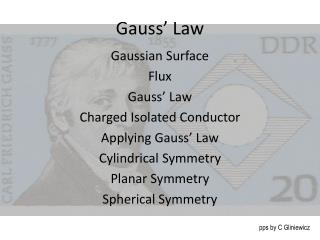DownloadDownload PresentationGauss’ Law

# Gauss’ Law

Download Presentation## Gauss’ Law

- - - - - - - - - - - - - - - - - - - - - - - - - - - E N D - - - - - - - - - - - - - - - - - - - - - - - - - - -
##### Presentation Transcript

1. Gauss’ Law Gaussian Surface Flux Gauss’ Law Charged Isolated Conductor Applying Gauss’ Law Cylindrical Symmetry Planar Symmetry Spherical Symmetry pps by C Gliniewicz

2. Symmetry is an important concept in physics. It allows some seemingly difficult problems to be solved with ease. Carl Friedrich Gauss imagined a hypothetical closed surface enclosing a charge distribution. This Gaussian surface can have any shape, but the shape that minimizes calculation of the electric field is one that mimics the symmetry of the charge distribution. A spherical group of charges would use a spherical surface. Gauss’ Law relates the electric field at points on the Gaussian surface to the net charge enclosed inside the surface. One can visualize the electric field as a distribution of arrow denoting the field. A Gaussian surface enclosing the charges will have these arrows piercing the surface. Some may be entering the surface. Others may be leaving the surface. As an analogy, one can visualize a screen in a river. Water passes through the screen in the same way. One can determine how much water goes through the screen by multiplying the speed of the water by the area of the screen. One then has the volume of water passing through the screen each second. This flow is called the flux from the latin word “to flow.” We always consider the flow perpendicular to the screen. The symbol used for flux is Φ. pps by C Gliniewicz

3. The flux of an electric field is just the electric field, perpendicular to a surface multiplied by the area of that surface. Note that area is a vector. The area is positive if it points out of the surface and is negative if it points into the surface. Since the flux has the sum of the electric field, it can be rewritten as an integral. The circle through the integral sign means that the calculation is done over a closed surface. When one thinks of the electric field as arrows, one can say that the flux is proportional to the number of lines passing through the Gaussian surface. To make calculation easier, try to pick a Gaussian surface which keeps the electric field lines perpendicular to the surface. For instance, for a point charge, a sphere with the point at the center would work nicely. A homogeneous field pointing in one direction might require a box-like surface with sides parallel to and perpendicular to the field or perhaps a cylindrical surface with the field perpendicular to the ends and parallel to the sides. pps by C Gliniewicz

4. Gauss’ Law, as mentioned earlier, relates the flux to the net charge enclosed inside the surface. If the enclosed charge is positive, the net flux will be positive, and if the enclosed charge is negative, the net flux will be negative. The electric field for a point charge is If one imagines a spherical surface around the charge at its center, all the radii are perpendicular to the surface which has a area, Substituting these quantities into Gauss’ Law gives It is obvious that the charged enclosed is equal to q, the charge causing the electric field. pps by C Gliniewicz

5. If an excess of charge is placed on an isolated conductor, that charge will move to the surface of the conductor and none of the charge will be found inside the conductor. The electric field inside the conductor must be zero since the presence of an electric field would create a force on any charge causing a flow of the free electrons in the conductor. An electric field would exist while the conductor is being charged, but would disappear once the charging halts. If a cavity were formed inside the conductor, there would no charge on the inner surface and no electric field. The electric field on the surface of a charged conductor depends on the charge per unit area. If a line of charge exists, one can create a Gaussian surface around the wire in the shape of a cylinder with the wire along its axis . pps by C Gliniewicz

6. If two charged plates lie parallel to one another with equal and opposite charges on the surfaces. The charges on each conducting plate will migrate to the facing sides because of attraction between the charges. The electric field between the two plates is constant and equal to If a charge is placed on a non-conducting sheet, the electric field is A shell of uniform charge attracts or repels a charged particle that is outside the shell as if all the shell’s charge were concentrated at the center of the shell. If the charged particle were inside the shell, no electric force would be experienced. If a non-conducting sphere has a charge distributed uniformly throughout the sphere and one desired to know the electric field at any point inside the sphere, one had to realize that any charge farther from the center of the sphere than the point of interest creates no electric field. Only the charge closer to the center create the electric field. Using r as the distance from the center and R as the radius of the sphere, and r≤R, one finds pps by C Gliniewicz